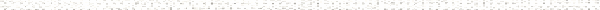A Precalculus Outline

 Chapter 7:  Hooked on ConicsObjective: The geometry of the Conic SectionsObjective: Graphing Circles in Standard Form> Objective: Completing the Square to put the equation of a Circle in Standard FormObjective: Finding the equation of a circleObjective: Distance formulaObjective: Midpoint formulaObjective: Graphing equationsObjective: Completing the squareObjective: Deriving the formula for a parabola with vertex at (0,0)Objective: Graphing ParabolasObjective: Completing the Square to put the equation of a Parabola in Standard FormObjective: Finding the focus and directrix of a parabolaObjective: Finding the equation of a parabolaObjective: Applications of ParabolasObjective: Distance formulaObjective: Midpoint formulaObjective: Graphing equationsObjective: Completing the squareObjective: Graphing EllipsesObjective: Completing the Square to put an Ellipse in Standard form to graphObjective: Finding the Equation of an ellipseObjective: Distance formulaObjective: Midpoint formulaObjective: Graphing equationsObjective: Completing the squareObjective: Graphing HyperbolasObjective: Finding the equation of a hyberbolaObjective: Completing the square to put the equation of a conic section in standard formObjective: Distance formulaObjective: Midpoint formulaObjective: Graphing equationsObjective: Completing the square